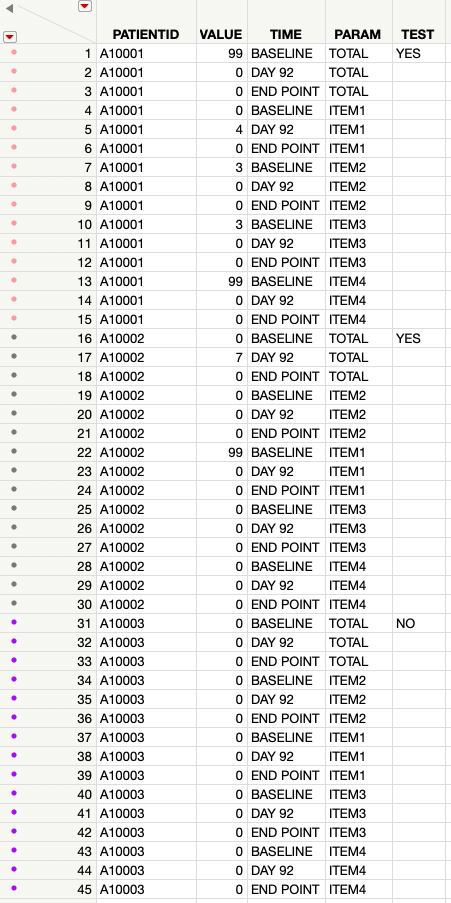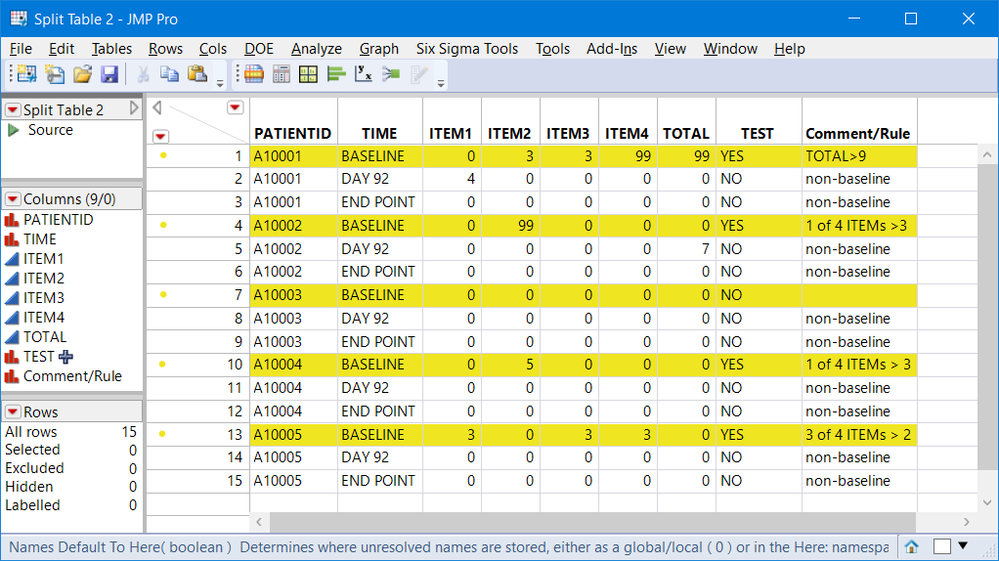The World Statistics Day celebration continues here in the Community. We all need reliable data for sound decision making. Do you have a data source that you trust most? Head over to Discussions to tell us about it.
Choose Language Hide Translation Bar
Highlighted

## Iterating Through Rows Question

Hello,

I'm so grateful for all of the previous replies, and am learning more and more about JSL every day. At this point, I am comfortable with the basics, and have learned about For loops, For Each Row and use of the Formula function. This was very helpful. As I mentioned in my previous post, I'm working on a dataset, and have 4 key columns:

1) PATIENTID: list of unique values for patient number
2) VALUE: Value of a patient rating at a particular timepoint
3) TIME: Timepoint at which patient rating was collected
4) PARAM: The patient rating is comprised of 4 individual items and a total score (ITEM1, ITEM2, ITEM3, ITEM4 and TOTAL). The total score is simply a sum of ITEM1+ITEM2+ITEM3+ITEM4.

From this, I'd like to create a 5th column (called "TEST"). The value for TEST is based on the following logic:

For an individual patient, if baseline values are:

• TOTAL > 9 or
• 1 of ITEM1, ITEM2, ITEM3 or ITEM4 are > 3 or
• 3 of ITEM1, ITEM2, ITEM3 or ITEM4 are > 2

Then the corresponding row in "TEST" should be evaluated as "YES". Non-baseline values, and other values should be evaluated as "NO". This logic should be iterated to every patient and every row in the datatable.

I'm having problems iterating through the rows in my dataset. Reason is that each patient has differing number of timepoints that they had filled out the scale. Some patients dropped out early. Since each patient has a different number of rows, I'm having difficulty figuring out how to iterate through the rows to write the script.

I've tried combining some IF statements, but get stuck at the point where I need to iterate to the next patient ID. I'm attaching a script that I wrote, but got stuck. Any help is greatly appreciated.

``````Names Default To Here( 1 );
dt = current data table ();
dt << New Column ("TEST", Character, Formula(

If(:TIME == "BASELINE",
theRow = Row();
thePatient = :PATIENTID;
If(:PARAM == "TOTAL",
If(N Rows(dt << Get Rows Where(
Row()>=theRow &
thePatient == :PATIENTID &
:VALUE > 9
)
) > 0
,"YES"
,"NO"
)))
));``````

Here is an example of what the data table looks like (real patient information redacted for privacy)1 ACCEPTED SOLUTION

Accepted Solutions
Highlighted

## Re: Iterating Through Rows Question

For an individual patient, if baseline values are:

• TOTAL > 9 or
• 1 of ITEM1, ITEM2, ITEM3 or ITEM4 are > 3 or
• 3 of ITEM1, ITEM2, ITEM3 or ITEM4 are > 2

Then the corresponding row in "TEST" should be evaluated as "YES". Non-baseline values, and other values should be evaluated as "NO". This logic should be iterated to every patient and every row in the datatable.

Complex rules in a stacked table can be done, but they get messy. It is much easier to manage if the table is unstacked by PATIENTID and TIME, where the rules are applied by PARAM. If the table is extremely large this method might not be efficient.

Attached is your example table with a two additional PATIENTID to test thes 3 rules.  Before using the attached script, you should check if the formula adequately captures your 3 rules. I added a comment column and colors to make it easier to check.Once split, here is the formula for the three rule TEST column. The script also includes the JSL code to unstack and reorganize the table.

``````dt_split:TEST << Set Each Value(
If(
(:TIME == "BASELINE") *
Maximum(
:TOTAL > 9,  //rule 1
Maximum( :ITEM1, :ITEM2, :ITEM3, :ITEM4 ) > 3, //rule 2
(:ITEM1 > 2) + (:ITEM2 > 2) + (:ITEM3 > 2) + (:ITEM4 > 2) >= 3 //rule 3
),
"YES",
"NO"
));
``````

.

4 REPLIES 4
Highlighted

## Re: Iterating Through Rows Question

For an individual patient, if baseline values are:

• TOTAL > 9 or
• 1 of ITEM1, ITEM2, ITEM3 or ITEM4 are > 3 or
• 3 of ITEM1, ITEM2, ITEM3 or ITEM4 are > 2

Then the corresponding row in "TEST" should be evaluated as "YES". Non-baseline values, and other values should be evaluated as "NO". This logic should be iterated to every patient and every row in the datatable.

Complex rules in a stacked table can be done, but they get messy. It is much easier to manage if the table is unstacked by PATIENTID and TIME, where the rules are applied by PARAM. If the table is extremely large this method might not be efficient.

Attached is your example table with a two additional PATIENTID to test thes 3 rules.  Before using the attached script, you should check if the formula adequately captures your 3 rules. I added a comment column and colors to make it easier to check.Once split, here is the formula for the three rule TEST column. The script also includes the JSL code to unstack and reorganize the table.

``````dt_split:TEST << Set Each Value(
If(
(:TIME == "BASELINE") *
Maximum(
:TOTAL > 9,  //rule 1
Maximum( :ITEM1, :ITEM2, :ITEM3, :ITEM4 ) > 3, //rule 2
(:ITEM1 > 2) + (:ITEM2 > 2) + (:ITEM3 > 2) + (:ITEM4 > 2) >= 3 //rule 3
),
"YES",
"NO"
));
``````

.

Highlighted

## Re: Iterating Through Rows Question

Thank you so much! This worked perfectly.

I'm reading through the script, and I was wondering if you could explain a little more. How does the MAXIMUM function work in this context? I understand that you're looking for a maximum of the 3 rules. However I see the * operator, which I assume means multiplication. I was confused on how the multiplication along with the MAXIMUM function work together in the IF statement.

Many thanks!
Highlighted

## Re: Iterating Through Rows Question

Your first post stated you how formulas and Set Each Value() iterates through the rows.  So here are a few pieces of information to keep in mind:

• Logical statements are evaluated as True or False. True is equivalent to 1 and False is equivalent to zero
• Suppose x is a numeric value. The JSL  function If( x, "YES", "NO" ) looks at the value of x. If x > 0, then the result is True(1)=>YES , otherwise False(0)=>NO.
• :TIME == "BASELINE" is a logical statement that returns a 1 or 0 so instead of using an "AND" (&), the multiply function will work. In other words, TIME must equal BASELINE and one of the rules must be true, i.e., 1*1 = 1. If either is false the result is zero or false.
• The outer Maximum() function has 3 arguments, the 3 rules. This maximum function replaces "OR" ( | ). If all 3 rules are false, the outer maximum returns a 0. If any one of the 3 rules returns a 1 (True) then the Maximum returns a 1. But keep in mind, this result has the logical multiplier so this only applies to BASELINE values.
• Regarding the rules: rule #1 ( :TOTAL > 9 ) returns a 1 or 0.  Rule #2 finds the maximum of the 4 items, if that is greater than 3, then at least one item is greater than 3. Rule #3  is the sum of 4 logical results (ITEM 1 >2, etc.) If that sum is greater than 2, then 3 or 4 of the items are greater than 2.

Using arithmetic on logical statements, makes it easier and faster to find cases that satisfy "k of n" rules. There are other methods like concatenate the  4 ITEMs and check if they are in { "0111", "1011", "1101", "1110"} or make them numeric and look for those values.  But the sum requires just one check (>2).  Since I was using arithmetic for rule #3, I used math functions, Maximum() instead of OR, and multiply instead of AND for the remaining logic.  Note you can have statements that mix logic functions with math functions.

Hope that helps.

Highlighted

## Re: Iterating Through Rows Question

Thank you, makes perfect sense now. Thanks for taking the time to explain, very helpful!!

Article Labels

There are no labels assigned to this post.